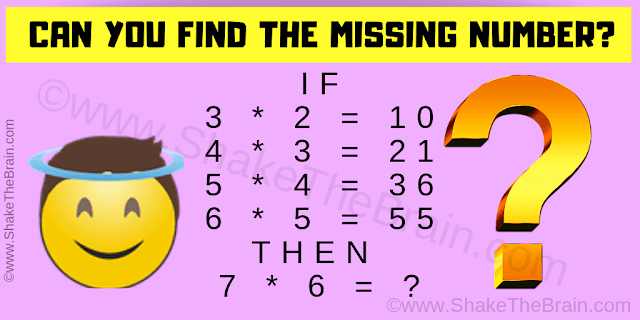How good you are in Mathematics? Here is the Math Logic Brain Teaser which will test your both Math skills as well as Logical reasoning power. In this brain teaser, some logical equations are given. Your challenge is to find the logic in these equations and then solve the last equation. Let us see how much time you will take to solve this equation?Can you find the missing number?
The answer to this Logic Math Brain Teaser can be viewed by clicking on the button. Please do give your best try before looking at the answer.